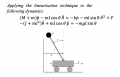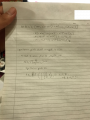# Equilibrium point and linear model

#### MrAl

Joined Jun 17, 2014
8,149
Hi,

Why dont you start by identifying all the variables. I can guess a few but would rather seem them all spelled out.

Joined Oct 5, 2018
34
ahh thank you sir! i think this seems to showcase a case of NEwtons second law where M and m is the masses of the equipment whereas p is to mimic restrictive forces? i.e friction? Unfortunately i am unsure of what j could be....

Additionally sir, what should be my approach to solving for equilibrium point? Do i apply Laplace transformation to the derivatives and equate them to zero? t

#### MrAl

Joined Jun 17, 2014
8,149
ahh thank you sir! i think this seems to showcase a case of NEwtons second law where M and m is the masses of the equipment whereas p is to mimic restrictive forces? i.e friction? Unfortunately i am unsure of what j could be....

Additionally sir, what should be my approach to solving for equilibrium point? Do i apply Laplace transformation to the derivatives and equate them to zero? t

Hi,

Well the only thing i can say at this point is that the eq point you are looking for will occur after all the dynamics have settled down. That could be at t approaching infinity for one. I'll have to look into this myself a little more before i can give any additional information.
Can you show us the complete lecture? That could help a lot here.
I was also hoping that a physical description would help us link p with angle.

Yes zero solution(s) are also called equilibrium points.

Last edited:

Joined Oct 5, 2018
34
ahhh, thank you sir for your reply! I was browsing through my lecture notes and saw the free-body diagram that my lecturer referenced to.I am still unsure of how to proceed with regards to the steps to find the equilibrium point and linear model. However, I am unsure of what my first step should be. Should I first change the derivatives through laplace? following that how do i proceed?

I am assuming equilibrium point is where left side equation is equal to the right side of the equation?

Thank you so much again for all your help and I look forward to solving the question with your help!

#### MrAl

Joined Jun 17, 2014
8,149
ahhh, thank you sir for your reply! I was browsing through my lecture notes and saw the free-body diagram that my lecturer referenced to.
View attachment 174387

I am still unsure of how to proceed with regards to the steps to find the equilibrium point and linear model. However, I am unsure of what my first step should be. Should I first change the derivatives through laplace? following that how do i proceed?

I am assuming equilibrium point is where left side equation is equal to the right side of the equation?

Thank you so much again for all your help and I look forward to solving the question with your help!

Hi,

Oh so it's the inverted pendulum cartThe way you could do this is to first linearize the trig functions. For example:
sin(a)=a (approximately)

and maybe:
cos(a)=1-sin(a)/20=1-a/20

They proceed maybe with state variables, you know that idea?

Joined Oct 5, 2018
34
Hi,

Oh so it's the inverted pendulum cartThe way you could do this is to first linearize the trig functions. For example:
sin(a)=a (approximately)

and maybe:
cos(a)=1-sin(a)/20=1-a/20

They proceed maybe with state variables, you know that idea?
Hi Sir, unfortunately i am not quite sure what you were saying. However, i found some references online that seemed to showcase them solving a similar case, albeit different constants. I have worked it out for the equilibrium point and have attached it here for your reference if you see anything wrong with it. I am still unsure of why the lecture question (sadly on my end) suddenly introduces a J variable which is not defined in the free body diagram.. weird....#### Attachments

Joined Oct 5, 2018
34
ahh additionally this is page 1 of the solution i solved!

#### MrAl

Joined Jun 17, 2014
8,149
Hi,

Sorry those pages are just too hard to read, i'll have to try to enhance them and see if i can read them.
But really you should type this stuff out.

Joined Oct 5, 2018
34
ahh most definitely sir.

i cannot attach my file here so i have attached my solution done on word for your reference.

#### Attachments

• 14.3 KB Views: 1

#### MrAl

Joined Jun 17, 2014
8,149
Hi,

Sorry i can not read docx files.
If you turn it into a pdf i can read it.

I forgot to mention, J is usually rotational inertia, and you can see the units are consistent with the addition in that factor (J+m*l^2).

What i could do is show a simpler example you can follow along with.
It looks like you could have the right basic idea though, replace p and theta and the like with state variables. And yes that leads to 4 equations.

Also, sometimes the mass m is taken to be much much smaller than M.
Also, around the equilib point sin and cos are often taken to be straight lines and that's part of the linearization. That happens because once equilib is reached the pendulum moves only slightly if the system is designed right and of course that's almost always a good assumptionAgain though if we can see the whole lecture that would help a lot.

Last edited:

#### MrAl

Joined Jun 17, 2014
8,149
Here is a worked example of the inverted cart pendulum.
The describing equations are a little simpler so the procedure is more clear.

Equations that describe the system:
 M*y2+m*l*a2-u(t)=0
 m*l*y2+m*l^2*a2-m*l*g*a=0

a is angle
a1 is first derivative of a
a2 is second derivative of a
y is forward movement of cart in same direction as u(t)
y1 is first derivative of y
y2 is second derivative of y

Assign state variables:
X1 = y
X2 = y1
X3 = a
X4 = a1

Replace y and a with state variables where:
x1 is time derivative of X1,
x2 is time derivative of X2,
x3 is time derivative of X3,
x4 is time derivative of X4,
(note the use of lower case for the time derivatives and upper case for the state variables)

and after doing that we get:
 M*x2+m*l*x4-u(t)=0
 x2+l*x4-g*X3=0

Now solve  for l*x4 and subst into  to get ,
and solve  for x2 and subst into  to get :
 g*m*X3+x2*M=u(t)
 g*M*X3-l*x4*M=u(t)

Solve for the time derivatives:
x1=X2
x2=u(t)/M-(g*m*X3)/M
x3=X4
x4=(g*X3)/l-u(t)/(l*M)

and from there you can solve in whatever manner you like.
Dont forget to observe the lower case and upper case variable meanings.
Also observe that lower case L looks like an upper case i.